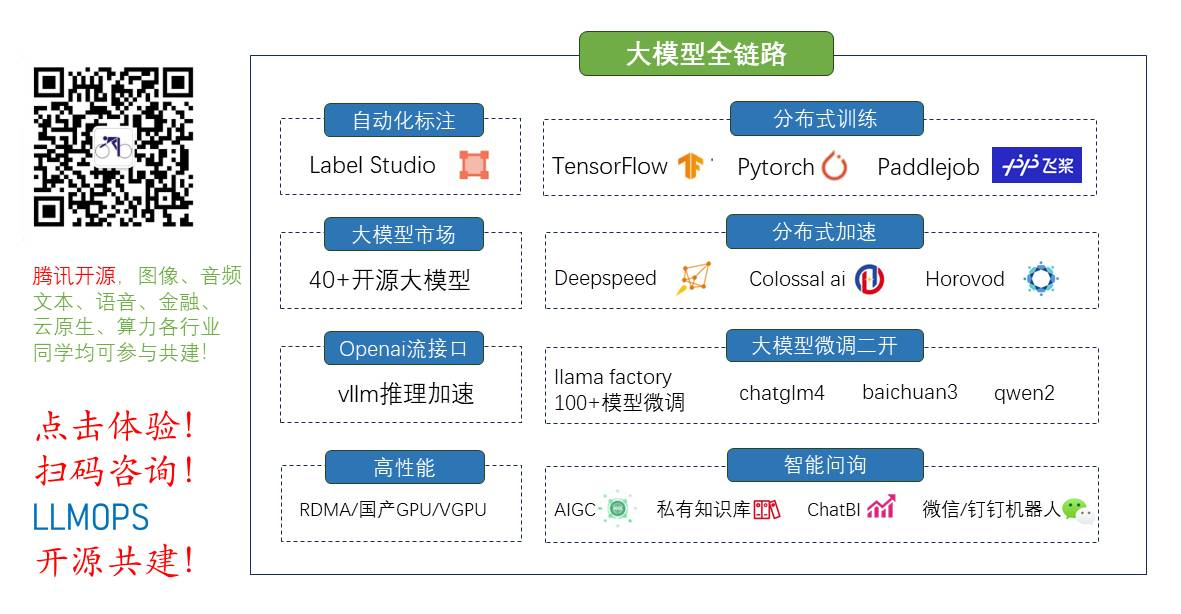• sin函数源码 MATLAB Notes by WENQI 该笔记记录本人在科研过程中所学到的MATLAB实用操作，为方便查阅总结如下，代码都经过本人验证实用，由于来源广泛，未能列全参考文献。 与系统交互 直接使用bat命令 system(&...
• sin函数源码 JavaPlot A Matlab上plot功能类似的Java实现，基于swing组件，方便Java绘制波形。 API说明 所有的绘图api均封装在Plot类下，使用和Matlab类似的函数名称。 figrue() 创建一个绘图面板 hold_on() 开启...
• sin函数源码 Pitch shifting User manual v1.0 初步完善pitch shifting的原理介绍及实现方式 Introduction Pitch shifting effect: 变调，移相效果器，该效果器可以实现对原始音频音调的调整，进行升调或者降调，...
• sin函数源码 Matplotlib 指南 作者 Nicolas P. Rougier 翻译 Sasasu Table of Contents 源代码在此处 所有的代码及素材遵守 . 你使用这个脚本 来测试 Matplotlib 是否安装成功。 本指南可在此处阅读 请一定也读一下 ...
• sin函数源码苍穹滴滴快的智能出行平台数据获取器 废弃项目与备份资源 目前所所爬取网站以关闭，故main.py不可用。 example.py可用于查看例子。 以下是一些以前爬取的数据（注意这些数据的正确性是受到质疑的， ） ...
• sin函数源码 Matlab_arrowPlot 提供一种Matlab绘制直线箭头以及非线性箭头的方式，可用以绘制坐标轴箭头。 该仓库包含两个Matlab函数： arrow.m：绘制任意两点间的直线箭头，可应用于二维或三维图形 arrowPlot.m：...
• sin函数源码 数模组新人指南 介绍及版权说明 数模组新生入门手册 —— 长期维护 > <（使用 GPL 许可证 非商用授权 如果使用其中内容请表明出处） 常用软件工具及基本配置方法（日常生活学习用） 系统软件 可以...
• ## matlab手写sin函数

千次阅读 2018-10-17 23:28:28
matlab手写sin函数 霍纳方法-计算多项式值 function y=nest(d,c,x,b) if nargin&lt;4 b=zeros(d,1); end y=c(d+1); for i=d:-1:1 y=y.*(x-b(i))+c(i); end Newton插值 function c=newtdd(x,y,n) for j=1...
matlab手写sin函数

霍纳方法-计算多项式值

function y=nest(d,c,x,b)
if nargin<4
b=zeros(d,1);
end
y=c(d+1);
for i=d:-1:1
y=y.*(x-b(i))+c(i);
end


Newton插值

function c=newtdd(x,y,n)

for j=1:n
v(j,1)=y(j);  %y column
end

for i=2:n  %column
for j=1:n+1-i   %row
v(j,i)=(v(j+1,i-1)-v(j,i-1))/(x(j+i-1)-x(j)); %count
end
end

for i=1:n
c(i)=v(1,i); %output the coefficient
end


sin函数

%create sin value
%input:x
%output:vagued sin(x)

function y=sin1(x)
%reserve the coefficient
b=pi*(0:3)/6;
yb=sin(b);
c=newtdd(b,yb,4);
s=1;
x1=mod(x,2*pi);
if x1>pi
x1=2*pi-x1;
s=-1;
end
if x1>pi/2
x1=pi-x1;
end
y=s*nest(3,c,x1,b);
end


测试

>> sin1(1)

ans =

0.8411

>> sin(1000)

ans =

0.8269

>> sin1(1000)

ans =

0.8263



展开全文• ## matlab:sin函数

千次阅读 2020-02-12 00:00:00
可以发现sin（pi）或者cos...由于sin，cos，tan等输入的参数为弧度制，而一般习惯角度制，所以解决的办法之一是用另外的函数，sind，cosd，tand等输入参数为角度制，就不会出现上述问题了。 sind(90) ans = 1 ...
可以发现sin（pi）或者cos（1/2*pi）不等于0，初步推测应该是浮点运算的精度问题。由于sin，cos，tan等输入的参数为弧度制，而一般习惯角度制，所以解决的办法之一是用另外的函数，sind，cosd，tand等输入参数为角度制，就不会出现上述问题了。

sind(90)
ans =  1


展开全文• matlab2c开发全解教程matlab2c调用方法：1、下载matlab2c动态链接库 2、将matlab2c.dll、matlab2c.lib和matlab2c.h放到项目头文件目录下 3、在cpp文件中引入下面的代码#include "Matlab2c.h" #pra全栈工程师开发手册 （作者：栾鹏）

matlab2c动态链接库下载matlab库函数大全matlab2c基础教程matlab2c开发全解教程
matlab2c调用方法：
1、下载动态链接库
2、将Matlab2c.dll拷贝到exe同目录下
3、将Matlab2c.h、Matlab2c.lib放到项目头文件目录下
4、在cpp文件中引入下面的代码
#include "Matlab2c.h"
#pragma comment(lib,"Matlab2c.lib")
using namespace Matlab2c;

matlab中sin函数简介
1、sin函数：

矩阵元素求sin值

2、用法说明

B =sin(A)返回矩阵元素求sin值

sin的c++源码实现
矩阵正弦函数，弧度为单位,sinf时c内部函数，
输入：矩阵a
输出：矩阵a的正弦值
Matrix Matlab2c::sin(Matrix& a)
{
int i,j;
Matrix p(a.row,a.column);
for (i=0;i<p.row;i++)
for (j=0;j<p.column;j++)
p(i,j) = std::sin(a(i,j));
return p;
}

sin函数的使用测试
#include "Matlab2c.h"
#pragma comment(lib,"Matlab2c.lib")
using namespace Matlab2c;

int main()
{
double a[]={PI/4,PI/5,PI/6,PI/7};
Matrix aa=Matrix(2,2,a);
Matrix cc=Matlab2c::sin(aa);
cout<<cc.toString()<<endl;

system("pause");
return 0;
}



展开全文c++
• 插值法实现sin函数： %calculate and print the sine function %input: x %output: sin(x) similar function y = sin2(x) %save a copy of x x_temp = x; %calculate the interpolation polynomial %save the ...
插值法实现sin函数：
%calculate and print the sine function
%input: x
%output: sin(x) similar
function y = sin2(x)
%save a copy of x
x_temp = x;
%calculate the interpolation polynomial
%save the coefficient
n = 4;
b = pi/4 + (pi/4)*cos((1:2:2*n-1)*pi/(2*n));
yb = sin(b);
c = newtdd(b, yb, n);c
%for any x, exchange it to base
%interpolation method calculation
len = size(x, 2);
s = ones(1, len);
y = zeros(1, len);
for i = 1 : len
if x(i) < 0
s(i) = s(i) * -1;
x(i) = x(i) * -1;
end
x1(i) =  mod(x(i), 2*pi);
if x1(i) > pi
x1(i) = 2*pi-x1(i);
s(i) = s(i) * -1;
end
if x1(i) > pi/2
x1(i) = pi - x1(i);
end
y(i) = s(i) * nest(n-1, c, x1(i), b);
end
plot(x_temp, y);
grid on
title('sin2(x)');
end


转载于:https://www.cnblogs.com/wsine/p/4634569.html
展开全文• ## Matlab 三角函数(sin)

万次阅读 2017-10-18 10:56:59
a='这是一个三角函数' t=0:0.01:2*pi; %从0到2pi，步长是0.01 y=sin(t); plot(t,y) %画坐标
• 脚本程序如下： ...%绘制出函数图像 x = 0:pi/1000:2*pi; %%定义x为0到2pi之间的一组向量，且x向量中相邻两个值之间的增量为pi/1000 y = sin(x); %z = cos(x); figure % 打开新的绘画窗口，可省略该...
• 使用函数 figure、axes、plot 代码 function shili01 h0=figure('toolbar' ,'none','position',[0,0,500,500],'name','实例01'); axes('parent' ,h0,'visible','...title('sin(x)函数曲线'); grid on 图形界面
• DDS原理： （1）将连续的正弦波信号离散化 （2）将离散化后的正弦波一个周期存储到ram中 ...sin_data = sin(2pin/N); %定点化 fix_sin_data = fix(sin_data*511);%取整 for i=1:N%将负数变为正数 if fix_sin_
• 使用递归算法 CORDIC 该模型生成给定角度的余弦和正弦函数。 您必须单击“Pulsa Aquí”块以放置角度并在模拟中建立最大误差。 只需解压缩文件
• matlab程序， bp bp网络逼近sin函数,值得一看，欢迎大家下载
• % 打开窗体钱先执行该函数 function gui_biancheng_OpeningFcn(hObject, eventdata, handles, varargin) handles.output = hObject; handles.x=-pi:0.01:pi; %全局变量 guidata(hObject, handle
• 软件：MatlabR2014a CodeBlock 1.新建个工程 2.输入程序，求得坐标并保存到txt文件中 3.用MATLAB建个M文件 4.输入绘图代码，如图 5.点击运行，M文件保存在新建工程所在的文件夹下 6.绘图完成 7...
• 软件：MatlabR2014a CodeBlock1.新建个工程2.输入程序，求得坐标并保存到txt文件中3.用MATLAB建个M文件4.输入绘图代码，如图5.点击运行，M文件保存在新建工程所在的文件夹下6.绘图完成7.如果想把图像以其他格式保存...
• %interp1对sin函数进行分段线性插值，调用interp1的时候，默认的是分段线性插值 y1=interp1(x,y,xx); figure plot(x,y,'o',xx,y1,'r') title('分段线性插值') %临近插值 y2=interp1(x,y,xx,'nearest'); figure plot...
• 函数代码，用于产生sin信号，是离散时间信号。 大家可以熟悉熟悉matlab的图形化界面。 MATLAB函数代码是自己编写的。数字信号处理入门级实验代码。 输入起始位置 用matlabGUI可以跑出来。比较简洁，希望对大家做...信号处理
• ## matlab三角函数用法

千次阅读 2019-07-29 22:05:54
matlab里三角函数sin,cos,tan,csc,sec和cot,是弧度制； 如，sin(pi/2)=1； sind,cosd,tand是角度制； 如，sind(90)=1； asin,acos,atan是弧度制反三角函数； asind,acosd,atand是角度制反三角函数； sinh,cosh,...
• x=0:2*pi;...%interp1对sin函数进行分段线性插值，调用interp1的时候，默认的是分段线性插值 y1=interp1(x,y,xx); figure plot(x,y,'o',xx,y1,'r') title('分段线性插值') %临近插值 y2=inter...
• (2)以弧度为单位：sin(pi/2) = 1 3.abs函数 求绝对值，复数的模，求字符串的ASCII码 4.取整 round:按照四舍五入规则取整 ceil：向上取整 floor：向下取整 fix：固定去靠近0的那个整数，舍去小数取整 5.求余数 例：m ......

# matlabsin函数matlab 订阅